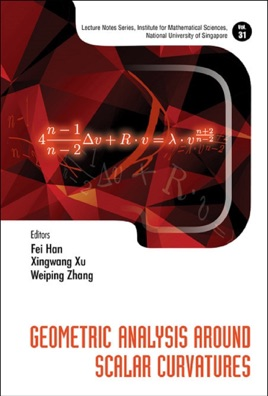• 42,99 €

## Description de l’éditeur

This volume contains three expanded lecture notes from the program Scalar Curvature in Manifold Topology and Conformal Geometry that was held at the Institute for Mathematical Sciences from 1 November to 31 December 2014. The first chapter surveys the recent developments on the fourth-order equations with negative exponent from geometric points of view such as positive mass theorem and uniqueness results. The next chapter deals with the recent important progress on several conjectures such as the existence of non-flat smooth hyper-surfaces and Serrin's over-determined problem. And the final chapter induces a new technique to handle the equation with critical index and the sign change coefficient as well as the negative index term. These topics will be of interest to those studying conformal geometry and geometric partial differential equations.
Contents:Lectures on the Fourth-Order Q Curvature Equation (Fengbo Hang and Paul C Yang)An Introduction to the Finite and Infinite Dimensional Reduction Methods (Manuel del Pino and Juncheng Wei)Einstein Constraint Equations on Riemannian Manifolds (Quôc Anh Ngô)